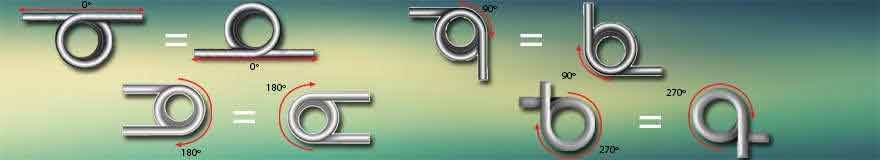<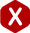# Free Position of a Torsion Spring Based on Total Coils

#### Definition:

The angle a torsion spring’s legs simulate when it is in its free position. This angle is determined by the total amount of coils this spring has since the legs start at the point where the coil ends. Also known as its unloaded position.

Determining the free position of a torsion spring based on its total coils is not an easy task. Here we will show you just how to do so. If you have a spring with 4.25 coils, for example, the free position would be of 90º (degrees). The reason for this is because when a spring has an solid number of coils such as 2, 3, 4, etc., the tips of the wire where the coil ended meet at the top of the spring. This causes your spring to have a 0º (degree) angle because the legs make a straight line.

If you want your torsion spring’s legs to be at an angle during its free state (without any load being applied to it), you will have to either add or subtract the fraction of a coil. Take a look at the following examples and formulas to learn more about how you calculate the torsional spring dimension of your torsion spring’s free position.

#### Example A.)

You have a spring with 4.25 coils. Since you have a “fraction” of a coil at the end of your torsion spring, you will calculate it as such and convert it into degrees by multiplying the partial last coil by 360º. This means you will multiply 0.250 by 360º as shown in the formula and diagram provided.

Fp = 360C

• Fp = Free Position
• C = Partial Coil
Fp = 360C
Fp = 360 (0.250)
Fp = 90º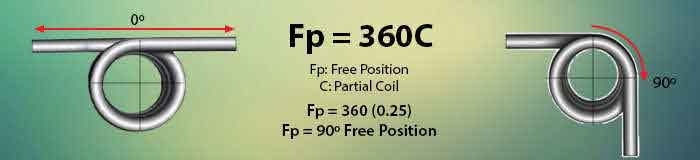#### Example B.)

Your mechanism needs your torsional spring to have a free position of 180º but how many coils does your spring need to have in order to achieve this free position? This time you will calculate backwards. Divide your free position of 180º by the 360º and you will get the fractional or partial coil you will need to add to your spring in order to achieve this free position.

C = Fp ÷ 360

• Fp = Free Position
• C = Partial Coil
C = Fp ÷ 360
C = 180 ÷ 360
C = 0.5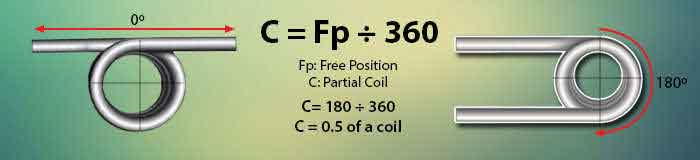The direction of wind of your torsional spring also has a lot to do with your spring’s free position and it will make a difference when being installed. When designing your torsion spring, you must take into consideration which leg will be stationary and which direction you expect the other leg to rotate or deflect. The legs will only be able to travel inward as shown in the deflection diagram provided below. To determine the direction of wind on a torsion spring, you must place it in a way to where you’re looking down the center of the inner diameter and the back leg must be horizontally on top. If the back leg is pointing to the left, it is a left hand torsion spring, if it is facing to the right, it is a right hand torsion spring. No matter which position your spring is in, the direction of wind will remain the same. Take a look at the images provided below to see some examples about how the free position affects your spring design and how to determine the direction of wind.

#### Determine Your Direction of Wind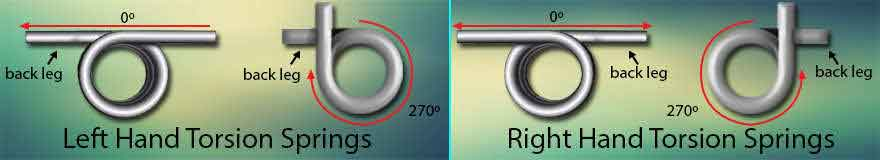#### Free Position Diagrams